# 1.6: Conversion From Decimal Numeration

Because octal and hexadecimal numeration systems have bases that are multiples of binary (base 2), conversion back and forth between either hexadecimal or octal and binary is very easy. Also, because we are so familiar with the decimal system, converting binary, octal, or hexadecimal to decimal form is relatively easy (simply add up the products of cipher values and place-weights). However, conversion from decimal to any of these “strange” numeration systems is a different matter.

The method which will probably make the most sense is the “trial-and-fit” method, where you try to “fit” the binary, octal, or hexadecimal notation to the desired value as represented in decimal form. For example, let’s say that I wanted to represent the decimal value of 87 in binary form. Let’s start by drawing a binary number field, complete with place-weight values:Well, we know that we won’t have a “1” bit in the 128’s place, because that would immediately give us a value greater than 87. However, since the next weight to the right (64) is less than 87, we know that we must have a “1” there.If we were to make the next place to the right a “1” as well, our total value would be 6410 + 3210, or 9610. This is greater than 8710, so we know that this bit must be a “0”. If we make the next (16’s) place bit equal to “1,” this brings our total value to 6410 + 1610, or 8010, which is closer to our desired value (8710) without exceeding it:By continuing in this progression, setting each lesser-weight bit as we need to come up to our desired total value without exceeding it, we will eventually arrive at the correct figure: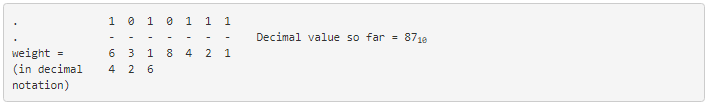This trial-and-fit strategy will work with octal and hexadecimal conversions, too. Let’s take the same decimal figure, 8710, and convert it to octal numeration:If we put a cipher of “1” in the 64’s place, we would have a total value of 6410 (less than 8710). If we put a cipher of “2” in the 64’s place, we would have a total value of 12810 (greater than 8710). This tells us that our octal numeration must start with a “1” in the 64’s place: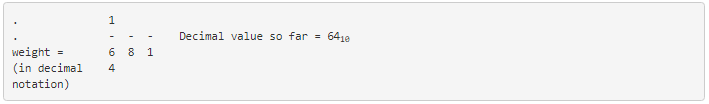Now, we need to experiment with cipher values in the 8’s place to try and get a total (decimal) value as close to 87 as possible without exceeding it. Trying the first few cipher options, we get:A cipher value of “3” in the 8’s place would put us over the desired total of 8710, so “2” it is!Now, all we need to make a total of 87 is a cipher of “7” in the 1’s place:Of course, if you were paying attention during the last section on octal/binary conversions, you will realize that we can take the binary representation of (decimal) 8710, which we previously determined to be 10101112, and easily convert from that to octal to check our work: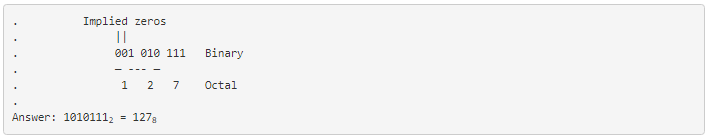Can we do decimal-to-hexadecimal conversion the same way? Sure, but who would want to? This method is simple to understand, but laborious to carry out. There is another way to do these conversions, which is essentially the same (mathematically), but easier to accomplish.

This other method uses repeated cycles of division (using decimal notation) to break the decimal numeration down into multiples of binary, octal, or hexadecimal place-weight values. In the first cycle of division, we take the original decimal number and divide it by the base of the numeration system that we’re converting to (binary=2 octal=8, hex=16). Then, we take the whole-number portion of division result (quotient) and divide it by the base value again, and so on, until we end up with a quotient of less than 1. The binary, octal, or hexadecimal digits are determined by the “remainders” left over by each division step. Let’s see how this works for binary, with the decimal example of 8710: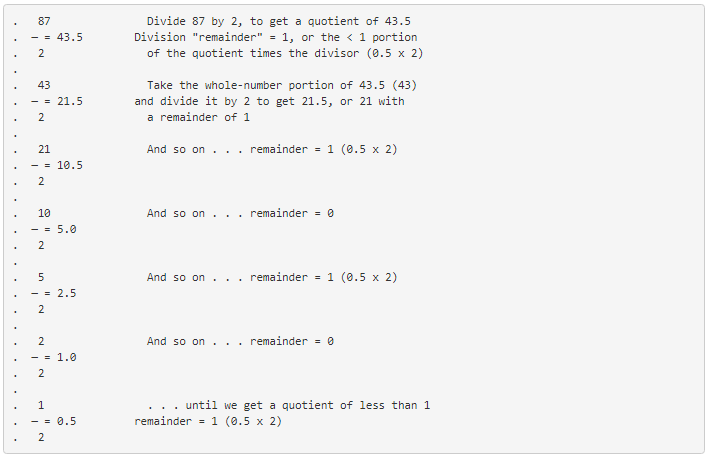The binary bits are assembled from the remainders of the successive division steps, beginning with the LSB and proceeding to the MSB. In this case, we arrive at a binary notation of 10101112. When we divide by 2, we will always get a quotient ending with either “.0” or “.5”, i.e. a remainder of either 0 or 1. As was said before, this repeat-division technique for conversion will work for numeration systems other than binary. If we were to perform successive divisions using a different number, such as 8 for conversion to octal, we will necessarily get remainders between 0 and 7. Let’s try this with the same decimal number, 8710: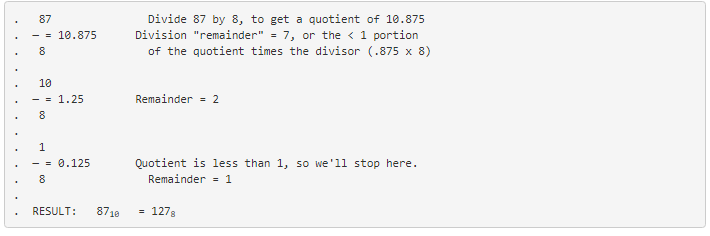We can use a similar technique for converting numeration systems dealing with quantities less than 1, as well. For converting a decimal number less than 1 into binary, octal, or hexadecimal, we use repeated multiplication, taking the integer portion of the product in each step as the next digit of our converted number. Let’s use the decimal number 0.812510 as an example, converting to binary:As with the repeat-division process for integers, each step gives us the next digit (or bit) further away from the “point.” With integer (division), we worked from the LSB to the MSB (right-to-left), but with repeated multiplication, we worked from the left to the right. To convert a decimal number greater than 1, with a < 1 component, we must use both techniques, one at a time. Take the decimal example of 54.4062510, converting to binary: